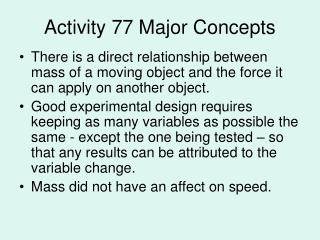DownloadDownload PresentationActivity 77 Major Concepts

# Activity 77 Major Concepts

Télécharger la présentation## Activity 77 Major Concepts

- - - - - - - - - - - - - - - - - - - - - - - - - - - E N D - - - - - - - - - - - - - - - - - - - - - - - - - - -
##### Presentation Transcript

1. Activity 77 Major Concepts • There is a direct relationship between mass of a moving object and the force it can apply on another object. • Good experimental design requires keeping as many variables as possible the same - except the one being tested – so that any results can be attributed to the variable change. • Mass did not have an affect on speed.

2. Activity 77 Analysis Questions • Did the mass of the cart affect the collision? Explain how you know this. Yes, the more massive the cart, the farther the block went. Explain further by citing your own results. • Why do you think mass did or did not have the effect you predicted? Since the distance the block moves is an indirect measurement of the force applied by the cart, this indicates that the more mass a moving object has, the greater the force it can apply during a collision.

3. What part(s) of the experiment design increase your confidence in the data? Using several trials and averaging the distance the block traveled tends to increase confidence by balancing out any errors. decrease your confidence in the data? The use of eyes only to determine exactly where the cart is released and how far the block moves is the most obvious design flaw.

4. If your car were in a collision, would you rather be hit by Vehicle 1 (2,027 kg) or Vehicle 2 (1,415 kg) from Activity 73? Explain. All other things being equal, it would be better to be hit by Vehicle 2 because the more massive Vehicle 1 has more potential to apply a greater force to a car.

5. Activity 78 Title: Force, Acceleration, and Mass

6. Read E-21 • Problem: What is the mathematical relationship between force, acceleration, and mass? • Hypothesis: Just answer the question. Do not use an if/then statement.

7. Review Acceleration: • What is acceleration? Acceleration occurs when an object speeds up, slows down, or changes direction. An object moving at constant speed and direction is not accelerating. You can also say acceleration is a change in motion.

8. Speed vs Acceleration • Although speed and acceleration are both rates that describe motion, they are not the same thing. Speed is the rate at which distance is traveled, whereas acceleration is the rate at which speed is changing. An object that has a larger rate of speeding up, as opposed to simply a high rate of speed, has a higher acceleration.

9. Example: • A car moving at a constant speed of 60 mph (no acceleration) • A car that moves from zero (0) to 20 mph in 5 seconds (acceleration of 4 mph).

10. What are the SI units for the following measurement? Copy into your notebook. Background information: • Speed Meters per second (m/s) • Mass Kilograms (kg) • Acceleration m/s2 (can be written as m/s /s, which is speed (m/s) divided by time (s) ). • Force newton (N)

11. newton (N) • The unit is named after Isaac Newton. One newton is about equal to the downward force (weight) of a 100-gram mass near the surface of Earth.

12. The Activity: • Thoroughly read the procedure on pgs E-22 to E-23. Set your notebook up as follows: Data: Part A: Comparing the Variables Follow procedure 1-3 and include all required information under the Part A heading (including analysis question 1). After most students have finish Part A, share with the class your answers to analysis #1.

13. After the class discussion, continue with parts B and C. Part B: Find the Equation procedure 4 Part C: Double-checking the Equation procedure 5 and 6

14. Discuss Results: • The equation, F = 2a works for the first set of data because this data set is based on a 2 kg mass. • It does not work for Experiment 2 in part C, which is based on a 4-kg mass. • “2” in the equation, F = 2a is the mass, which would have to be a 4 in Experiment 2.

15. The generalized equation which works for both is: F = ma The relationship can be written in 3 ways: F = ma a = m = F m F a This relationship between force, acceleration and mass is known as Newton’s Second Law Write all this in your data section

16. For the rest of the period: • Watch a Bill Nye video on motion. Answer the questions on the sheet provided. It will be turned in for a grade. • Do Activity 78, analysis 1-5 Give thorough answers to your questions and compare your answers with your group. • Read pg. 350-355 in PH and answers question 1-5 on pg. 355 on separate paper.

17. Homework: • Activity 78, Analysis questions 1-5 • Finish reading pg. 350-355 in PH and answer questions 1-5 on pg. 355